# Examples¶

Notebooks examples can be found on:

• Binder. This allows you to run and modify the notebooks.

## Spatial coverages¶

Here are some code examples manipulating `MOC` objects.

This example:

• Load the coverage of SDSS from a FITS file.

• Plot the MOC by:

1. Defining a matplotlib figure.

2. Defining an astropy WCS representing the field of view of the plot.

3. Call the `mocpy.moc.MOC.fill()` and `mocpy.moc.MOC.border()` so that mocpy plot on a matplotlib axis.

4. Set the axis labels, a title, enable the grid and plot the final figure.

```from mocpy import MOC, World2ScreenMPL
from astropy.coordinates import Angle, SkyCoord
import astropy.units as u
filename = './../../resources/P-SDSS9-r.fits'
moc = MOC.from_fits(filename)
# Plot the MOC using matplotlib
import matplotlib.pyplot as plt
fig = plt.figure(111, figsize=(15, 10))
# Define a astropy WCS easily
with World2ScreenMPL(fig,
fov=200 * u.deg,
center=SkyCoord(0, 20, unit='deg', frame='icrs'),
coordsys="icrs",
rotation=Angle(0, u.degree),
projection="AIT") as wcs:
ax = fig.add_subplot(1, 1, 1, projection=wcs)
# Call fill with a matplotlib axe and the `~astropy.wcs.WCS` wcs object.
moc.fill(ax=ax, wcs=wcs, alpha=0.5, fill=True, color="green")
moc.border(ax=ax, wcs=wcs, alpha=0.5, color="black")
plt.xlabel('ra')
plt.ylabel('dec')
plt.title('Coverage of P-SDSS9-r')
plt.grid(color="black", linestyle="dotted")
plt.show()
```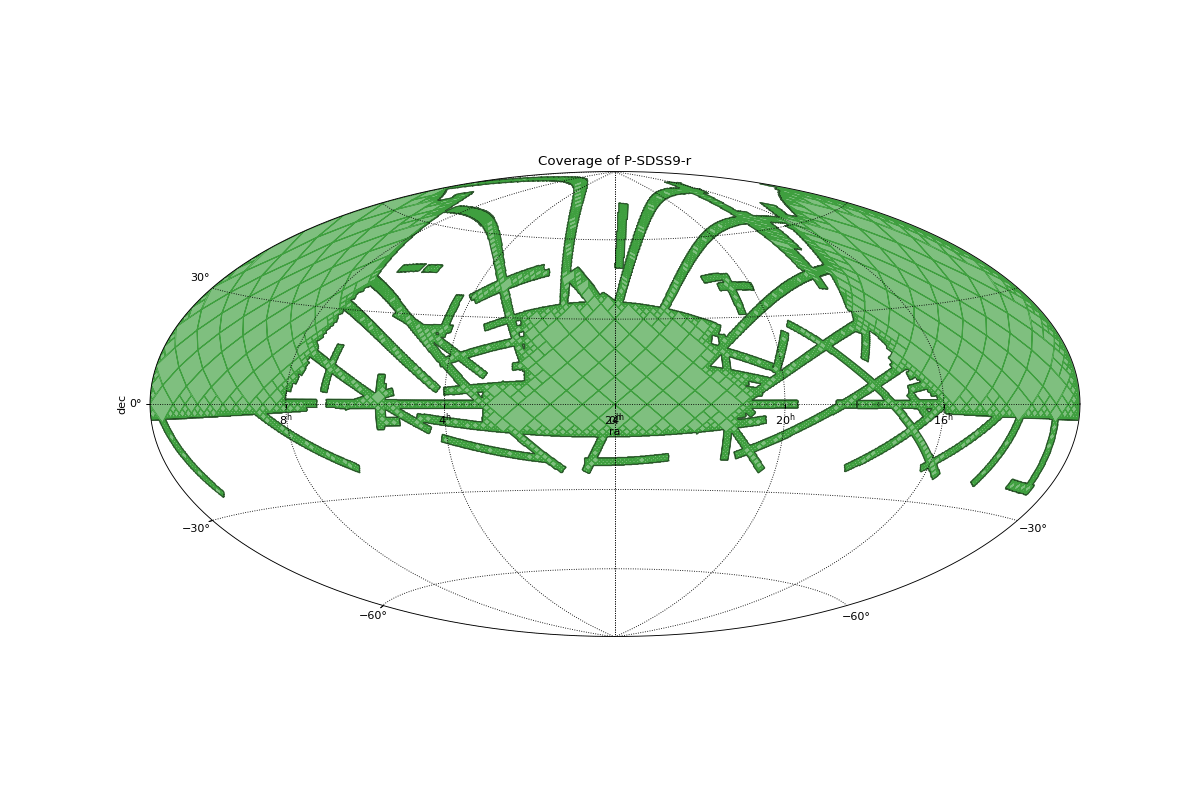### Intersection between GALEX and SDSS¶

This example:

• Load the coverages of SDSS and GALEX from FITS files.

• Compute their intersection

• Compute their union

• Plot the resulting intersection and union on a same matplotlib axis.

```from mocpy import MOC, World2ScreenMPL
from astropy.coordinates import Angle, SkyCoord
import astropy.units as u
sdss = MOC.from_fits('./../../resources/P-SDSS9-r.fits')
galex = MOC.from_fits('./../../resources/P-GALEXGR6-AIS-FUV.fits')
# Compute their intersection
inter = sdss.intersection(galex)
union = sdss.union(galex)
# Plot the MOC using matplotlib
import matplotlib.pyplot as plt
fig = plt.figure(111, figsize=(10, 10))
# Define a astropy WCS easily
with World2ScreenMPL(fig,
fov=160 * u.deg,
center=SkyCoord(0, 0, unit='deg', frame='icrs'),
coordsys="icrs",
rotation=Angle(0, u.degree),
projection="AIT") as wcs:
ax = fig.add_subplot(1, 1, 1, projection=wcs)
# Call fill with a matplotlib axe and the `~astropy.wcs.WCS` wcs object.
union.fill(ax=ax, wcs=wcs, alpha=0.5, fill=True, color="red", linewidth=0, label="Union")
union.border(ax=ax, wcs=wcs, alpha=1, color="red")

inter.fill(ax=ax, wcs=wcs, alpha=0.5, fill=True, color="green", linewidth=0, label="Intersection")
inter.border(ax=ax, wcs=wcs, alpha=1, color="green")
ax.legend()

plt.xlabel('ra')
plt.ylabel('dec')
plt.title('Logical operations between SDSS and GALEX')
plt.grid(color="black", linestyle="dotted")
plt.show()
```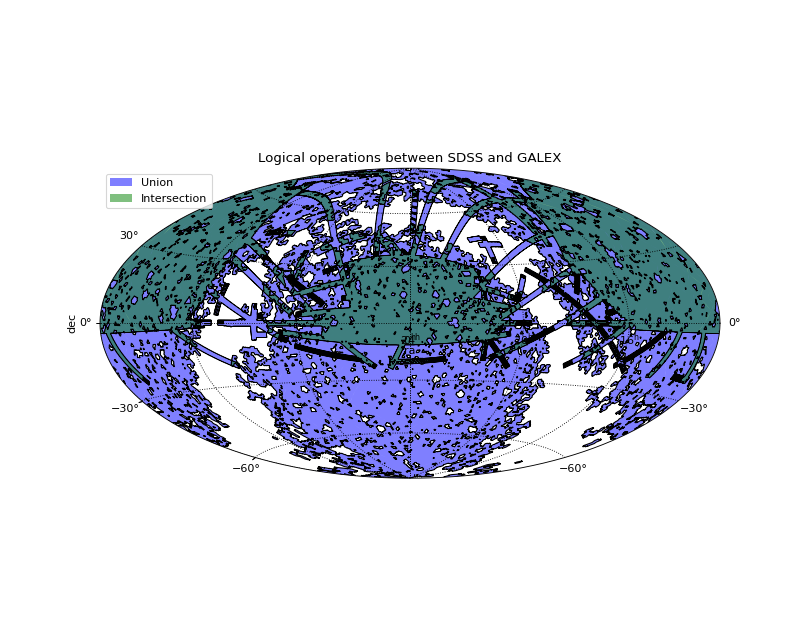### Create a MOC from a concave polygon¶

This example shows how to call `mocpy.moc.MOC.from_polygon()` or `mocpy.moc.MOC.from_polygon_skycoord()`.

```from mocpy import MOC, World2ScreenMPL
from astropy.coordinates import Angle, SkyCoord
import astropy.units as u

import numpy as np
# The set of points delimiting the polygon in deg
vertices = np.array([[18.36490956,  5.        ],
[15.7446692 ,  6.97214743],
[16.80755056, 10.29852928],
[13.36215502, 10.14616136],
[12.05298691, 13.10706197],
[ 9.54793022, 10.4556709 ],
[ 8.7677627 ,  7.80921809],
[ 9.71595962,  5.30855011],
[ 7.32238541,  6.44905255],
[ 0.807265  ,  6.53399616],
[ 1.08855146,  3.51294225],
[ 2.07615384,  0.7118289 ],
[ 3.90690158, -1.61886929],
[ 9.03727956,  2.80521847],
[ 9.22274427, -4.38008174],
[10.23563378,  4.06950324],
[10.79931601,  3.77655576],
[14.16533992,  1.7579858 ],
[19.36243491,  1.78587203],
[15.31732084,  5.        ]])
skycoord = SkyCoord(vertices, unit="deg", frame="icrs")
# A point that we say it belongs to the inside of the MOC
inside = SkyCoord(ra=10, dec=5, unit="deg", frame="icrs")
moc = MOC.from_polygon_skycoord(skycoord, max_depth=9)
moc.write('polygon_moc.fits', format='fits', overwrite=True)
# Plot the MOC using matplotlib
import matplotlib.pyplot as plt
fig = plt.figure(111, figsize=(10, 10))
# Define a astropy WCS easily
with World2ScreenMPL(fig,
fov=20 * u.deg,
center=SkyCoord(10, 5, unit='deg', frame='icrs'),
coordsys="icrs",
rotation=Angle(0, u.degree),
# The gnomonic projection transforms great circles into straight lines.
projection="TAN") as wcs:
ax = fig.add_subplot(1, 1, 1, projection=wcs)
# Call fill with a matplotlib axe and the `~astropy.wcs.WCS` wcs object.
moc.fill(ax=ax, wcs=wcs, alpha=0.5, fill=True, color="red", linewidth=1)
moc.border(ax=ax, wcs=wcs, alpha=1, color="red")

plt.xlabel('ra')
plt.ylabel('dec')
plt.title('MOC from a polygon')
plt.grid(color="black", linestyle="dotted")
plt.show()
```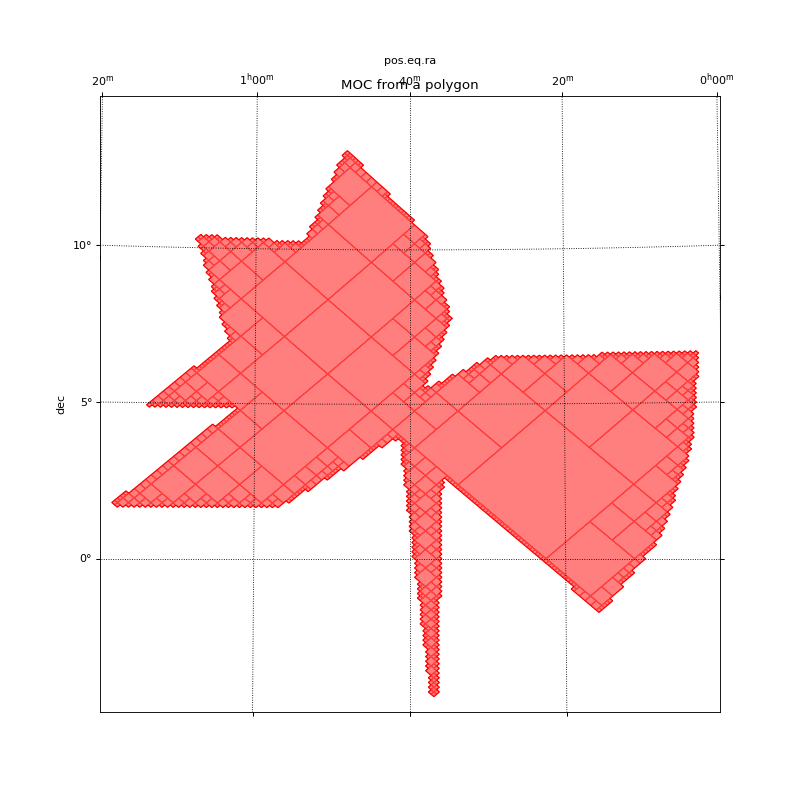### Get the border(s) of a MOC¶

This example shows how to call `mocpy.moc.MOC.get_boundaries()`. The borders are returned as a list of `SkyCoord` each defining one border. In this example:

1. The sky coordinates defining the border of the MOC are projected to the pixel image system.

2. Then, a matplotlib path is defined from the projected vertices.

3. Finally the path is plot on top of the MOC.

```from mocpy import MOC, World2ScreenMPL
from astropy.coordinates import SkyCoord, Angle

from matplotlib.path import Path
from matplotlib.patches import PathPatch

import astropy.units as u

moc = MOC.from_fits('polygon_moc.fits')
skycoords = moc.get_boundaries()

# Plot the MOC using matplotlib
import matplotlib.pyplot as plt
fig = plt.figure(111, figsize=(10, 10))
# Define a astropy WCS easily
with World2ScreenMPL(fig,
fov=20 * u.deg,
center=SkyCoord(10, 5, unit='deg', frame='icrs'),
coordsys="icrs",
rotation=Angle(0, u.degree),
# The gnomonic projection transforms great circles into straight lines.
projection="TAN") as wcs:
ax = fig.add_subplot(1, 1, 1, projection=wcs)
# Call fill with a matplotlib axe and the `~astropy.wcs.WCS` wcs object.
moc.fill(ax=ax, wcs=wcs, alpha=0.5, fill=True, color="red", linewidth=1)
moc.border(ax=ax, wcs=wcs, alpha=1, color="red")

# Plot the border
from astropy.wcs.utils import skycoord_to_pixel
x, y = skycoord_to_pixel(skycoords, wcs)
p = Path(np.vstack((x, y)).T)
patch = PathPatch(p, color="black", fill=False, alpha=0.75, lw=2)

plt.xlabel('ra')
plt.ylabel('dec')
plt.title('Get the border(s) of a MOC')
plt.grid(color="black", linestyle="dotted")
plt.show()
```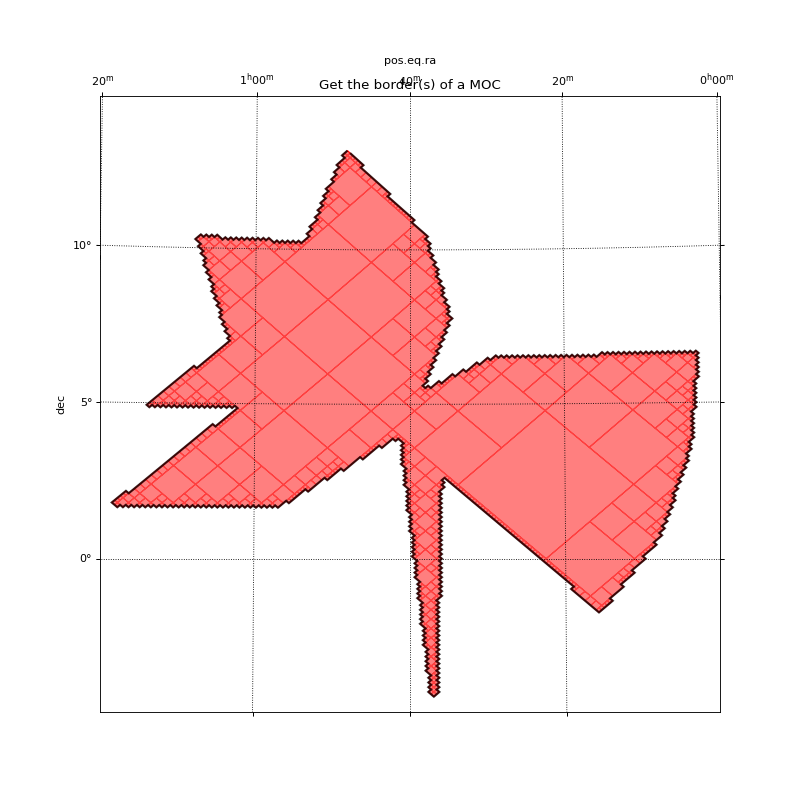### Gravitational Waves MOCs¶

This example shows the probability confidence regions of gravitational waves. HEALPix cells are given under the uniq pixel notation. Each pixel is associated with a specific value. We can create a MOC from which a GW has x% of change of being localized in it. By definition the MOC which has 100% of chance of containing a GW is the full sky MOC.

```from astropy.io import fits
fits_image_filename = './../../resources/bayestar.multiorder.fits'

with fits.open(fits_image_filename) as hdul:
hdul.info()
hdul.columns

data = hdul.data

uniq=data['UNIQ']
probdensity=data['PROBDENSITY']

import astropy_healpix as ah
import astropy.units as u

level, ipix = ah.uniq_to_level_ipix(uniq)

prob = probdensity * area

from mocpy import MOC

import numpy as np
cumul_to = np.linspace(0.5, 0.9, 5)[::-1]
colors = ['blue', 'green', 'yellow', 'orange', 'red']
mocs = [MOC.from_valued_healpix_cells(uniq, prob, cumul_to=c) for c in cumul_to]

from mocpy import World2ScreenMPL
from astropy.coordinates import Angle, SkyCoord
import astropy.units as u
# Plot the MOC using matplotlib
import matplotlib.pyplot as plt
fig = plt.figure(111, figsize=(15, 10))
# Define a astropy WCS easily
with World2ScreenMPL(fig,
fov=50 * u.deg,
center=SkyCoord(315, 15, unit='deg', frame='icrs'),
coordsys="icrs",
rotation=Angle(0, u.degree),
projection="AIT") as wcs:
ax = fig.add_subplot(1, 1, 1, projection=wcs)
# Call fill with a matplotlib axe and the `~astropy.wcs.WCS` wcs object.
for (moc, c, col) in zip(mocs, cumul_to, colors):
moc.fill(ax=ax, wcs=wcs, alpha=0.5, linewidth=0, fill=True, color=col, label='confidence probability ' + str(round(c*100)) + '%')
moc.border(ax=ax, wcs=wcs, alpha=0.5, color=col)

ax.legend()

plt.xlabel('ra')
plt.ylabel('dec')
plt.title('Bayestar')
plt.grid(color="black", linestyle="dotted")
plt.show()
```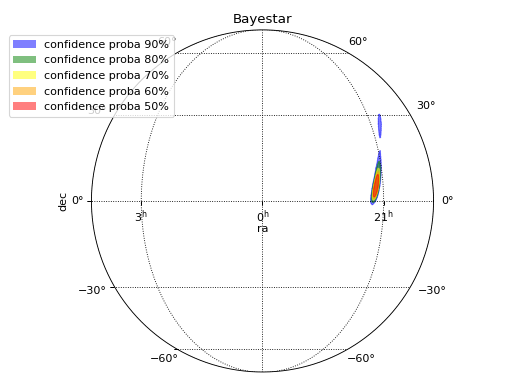### Performing computation on the pixels of an FITS image lying in a MOC¶

This example shows how a MOC can filter pixels from a specific FITS image (i.e. associated with a WCS). These pixels can then be retrieved from the image for performing some computations on them: e.g. mean, variance analysis thanks to numpy/scikit-learn…

```from astropy.io import fits
from astropy.wcs import WCS as WCS
from mocpy import MOC
from astropy.visualization import simple_norm
import astropy.units as u
import numpy as np

# load 2MASS cutout covering the galactic plane

moc = MOC.from_fits('http://skies.esac.esa.int/Spitzer/IRAC1_bright_ISM/Moc.fits')

# create WCS from 2MASS image header

# compute skycoords for every pixel of the image

xv, yv = np.meshgrid(np.arange(0, width), np.arange(0, height))
skycoords = twomass_wcs.pixel_to_world(xv, yv)

ra, dec = skycoords.icrs.ra.deg, skycoords.icrs.dec.deg
# Get the skycoord lying in the MOC mask
mask_in_moc = moc.contains(ra * u.deg, dec * u.deg)

img = hdu.data
img_test = img.copy()

# Set to zero the pixels that do not belong to the MOC

# Plot the filtered pixels image
import matplotlib.pyplot as plt
fig = plt.figure(111, figsize=(15, 10))
ax = fig.add_subplot(1, 1, 1, projection=twomass_wcs)

im = ax.imshow(img_test, origin='lower', norm=simple_norm(hdu.data, 'sqrt'), vmin=-1, vmax=150)
plt.show()
```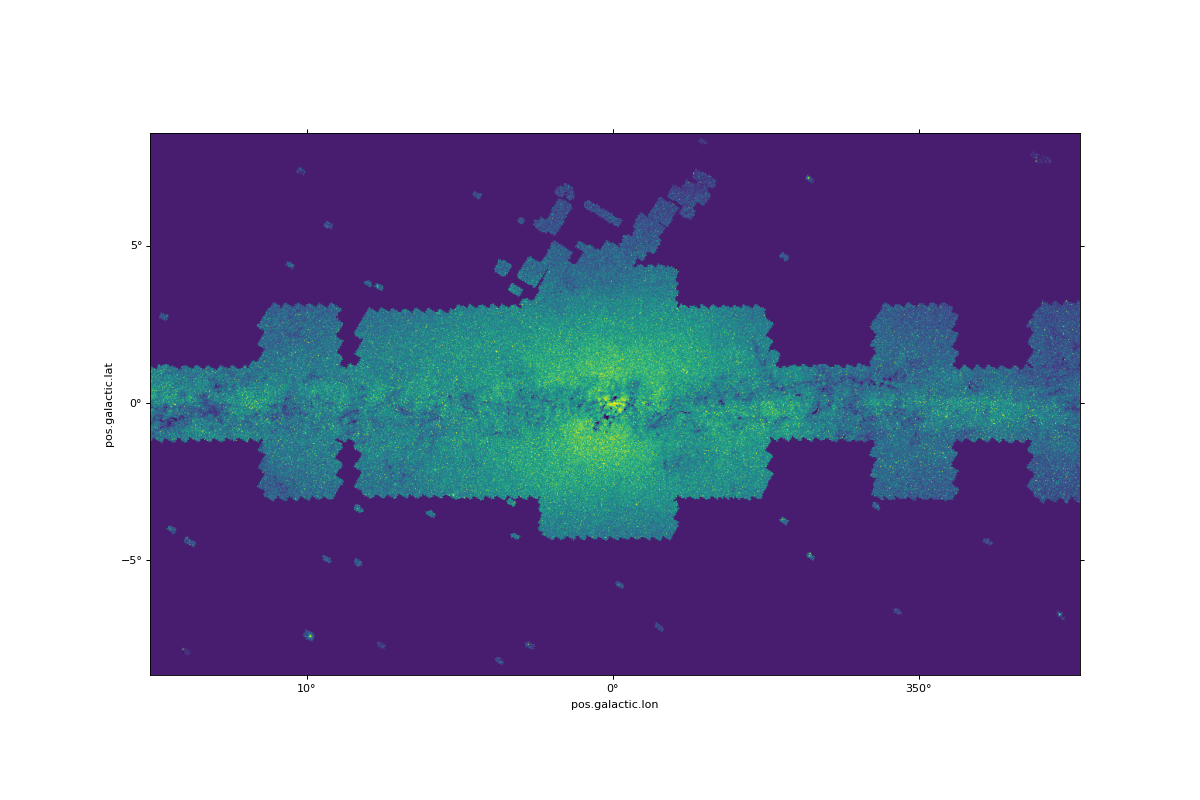```# Sum of pixels lying in the MOC

# Sum of pixels of the whole image
np.sum(img)
```

## Temporal coverages¶

A class `TimeMOC` describes temporal coverages.

Please refer to the following notebook here for how to use it.

## Space & Time coverages¶

Space-Time coverages are a new feature of `mocpy` since its version 0.7.0 and are an attempt initiated by the Virtual Observatory for binding spatial and temporal coverages together. See its description formalized by the IVOA here.

Space-Time coverages allows you to:

1. Retrieve the spatial coverage observed by a mission within a set of time frames (i.e. `Time` ranges).

2. Retrieve the temporal coverage observed by a mission within a spatial coverage.

As we do for spatial or temporal coverages, one can also perform the union, intersection or difference between two Space-Time coverages.

Please refer to the following notebook here for how to compute and query Space-Time coverages.